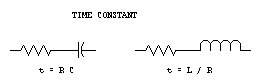TIME CONSTANT

Introduction | Questions | Quiz
 INTRODUCTION In RC (resistive & capacitive) circuits, time constant is the time in seconds required to charge a capacitor to 63.2% of the applied voltage. This period is referred to as one time constant. After two time constants, the capacitor will be charged to 86.5% of the applied voltage. The same time constant applies for discharging a capacitor through a resistor. After one time constant, a capacitor will have discharged to (100 - 63.2) 36.8% of the initial stored charge. Formula: t = RC t = time constant in seconds R = resistance in ohms C = capacitance in farads Example: The time constant for a circuit having a 100 microfarad capacitor in series with a 470K resistor is: .0001 * 470 000 = 47 seconds In RL (resistive & inductive) circuits, time constant is the time in seconds required for current to build up to 63.2% of the maximum current. This period is referred to as one time constant. Current in an inductive circuit does not immediately reach maximum value due to the back EMF (electromotive force) created by the change in current. Back EMF opposes the applied EMF A-001-001-001.... What is the meaning of the term "time constant" in an RL circuit? the time required for the current in the circuit to build up to 36.8% of the maximum value the time required for the voltage in the circuit to build up to 63.2% of the maximum value the time required for the voltage in the circuit to build up to 36.8% of the maximum value the time required for the current in the circuit to build up to 63.2% of the maximum value A-001-001-002.... What is the term for the time required for the capacitor in an RC circuit to be charged to 63.2% of the supply voltage? an exponential rate of one one time constant a time factor of one one exponential period A-001-001-003.... What is the term for the time required for the current in an RL circuit to build up to 63.2% of the maximum value? one time constant an exponential period of one a time factor of one one exponential rate A-001-001-004.... What is the term for the time it takes for a charged capacitor in an RC circuit to discharge to 36.8% of its initial value of stored charge? a discharge factor of one an exponential discharge of one one time constant one discharge period A-001-001-005.... What is meant by "back EMF" ? a current that opposes the applied EMF a voltage that opposes the applied EMF an opposing EMF equal to R times C percent of the applied EMF a current equal to the applied EMF A-001-001-006.... After two time constants, the capacitor in an RC circuit is charged to what percentage of the supply voltage? 63.2% 86.5% 95% 36.8% A-001-001-007.... After two time constants, the capacitor in an RC circuit is discharged to what percentage of the starting voltage? 13.5% 36.8% 86.5% 63.2% A-001-001-008.... What is the time constant of a circuit having a 100 microfarad capacitor in series with a 470 kilohm resistor? 4700 seconds 470 seconds 0.47 seconds 47 seconds A-001-001-009.... What is the time constant of a circuit having a 470 microfarad capacitor in series with a 470 kilohm resistor? 221 000 seconds 47 000 seconds 221 seconds 470 seconds A-001-001-010.... What is time constant of a circuit having a 220 microfarad capacitor in series with a 470 kilohm resistor? 470 000 seconds 470 seconds 103 seconds 220 seconds QUIZ Try the QUIZ for this topic.HAMSTUDY.COM
Webmaster: Frank VanderZande - VE7AV